# Hello DeepQ

In this document I'll describe my first attempt at Deep Q Learning (DQL) on the openai gym project. I'll attempt to explain some theory and I'll demonstrate where my approach needs some extra work. It is by no means written by an expert and it is likely that I've made a small error in maths by applying the laymans maths a bit too much.

All the code for this can be found on github.

## The Problem

The cartpole problem is a classic one; given a small cart you need to balance a pole on it.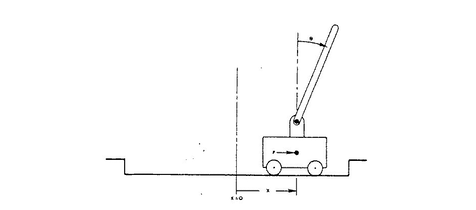There's a solution that involves physics but instead of doing all the maths ourselves we'd like to design a robot that can instead learn to balance the pole. We're given an environment that will let us know if the pole is still balanced and some controls that we can play with. It will be the robots job to understand the environment enough such that it can learn how to balance the pole.

Part of the problem lies in the fact that only after the pole has fallen we'll learn how well the robot has performed. We don't know if the pole fell because of some decision that was made in the beginning or if this was due to some decision made at the end of the trial. This makes part of the problem hard, we cannot just blindly apply machine learning or operations research methods because the system doesn't directly allocate reward to choices that are made.

Another part of the problem is that we'd like to have a general way that doesn't solve just this problem, but many problems. Given some environment, can we have an algorithm that designs the agent for us, without needing us (humans) to understand the environment beforehand. If we're able to do that, then robots truely become professional companions.

## Q-Learning

A popular method of looking at these sorts of problems is to consider Q-learning. We try to figure out the value that is obtained given some state of the environment $$S$$ and some action of the agent $$a$$. The value of picking an action $$a$$ from a state $$S_t$$ is defined via;

$Q(S_t, a) = R(S_t, a) + \gamma Q(S_{t+1}, a^*)$

The main observation here is the recursion.

$$R(S_t, a)$$ is the short term reward that we get from picking action $$a$$. By looking at this function you may realize a modelling choice. It tells us that the reward of picking an action from a certain state can be split up into two different pieces: a short term reward and value of being in a new state $$S_{t+1}$$. You'll also notice a parameter $$\gamma$$ which is used to discount future values.

The value of being in a state $$S_{t+1}$$ is dependant on the optimal action from that state. This action may be probibalistic but we assume that there is some optimal such that we can deduct value of being in a state $$S_{t+1}$$.

#### An example

I can imagine the math might distract from the understanding. So I'll include a simple example to demonstrate how the values are assigned and logged.

We're working with the cartpole. We start an instance of the environment (let's call this the game). The game ends if the pole seems to be falling beyond repair. There are two inputs; left and right. The environment gives us some state $$S_t$$ back. Let's assume a very basic game where we only witnessed 4 states; $$A$$, $$B$$, $$C$$ and $$D$$. In this case $$D$$ is the end state, when the pole is falling beyond repair.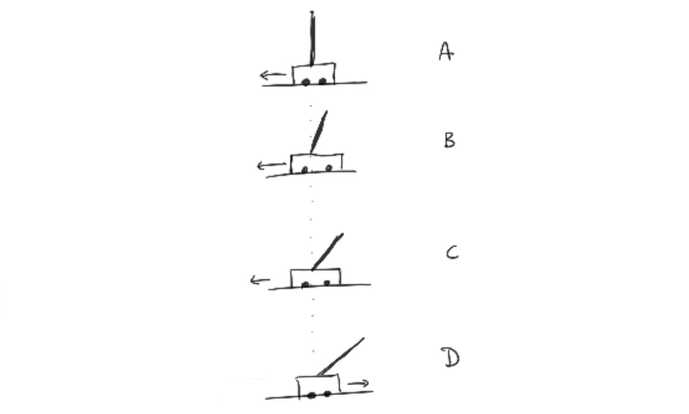We have lots of $$(S,a)$$ pairs now: $$(A, \leftarrow)$$, $$(B, \leftarrow)$$, $$(C, \leftarrow)$$ and $$(D, \rightarrow)$$. We now need to assign value to it.

The short term reward we get from the system is 1 for every timestep that we survive. This includes the final timestep, so the function that describes the measured value $$v$$ can be described via;

$v(D, \rightarrow) = R(S_t, a) = 0$

This is because in the final step, there is not further step and thus we get a value of zero. For all the other states $$R(S_t, a) = 1$$. Let's say we've got a discount rate equal to $$\gamma = 0.9$$. We can then propagate the value for the timestep before;

$v(C, \leftarrow) = 1 + \gamma v(D, \rightarrow) = 1$

This results moves recursively, such that;

$v(B, \leftarrow) = 1 + \gamma v(C, \leftarrow) = 1 + \gamma$

And such that;

$v(A, \leftarrow) = 1 + \gamma v(B, \leftarrow) = 1 + \gamma(1 + \gamma)$

These values all depend on the choice of $$\gamma$$. Assuming $$\gamma = 0.9$$ we end up with;

$v(D, \rightarrow) = 0  v(C, \leftarrow) = 1  v(B, \leftarrow) = 1.9  v(A, \leftarrow) = 2.71$

By repeating these steps, we can create a set of training data containing triplets of states, actions and values: $$(S, a, v)$$. The goal is to use these datapoints to come up with some model that can describe $$Q(S_t, a)$$ for us such that we can define a policy to pick the best action. One blunt interpretation of this is to estimate;

$Q(S_t, a) = \mathbb{E}[V(S_t, a)]$

## Using a Neural Network

We now know how to get a list of $$(S, a, v)$$ triplets via trial and error. The next step is to find a way to predict this. Turns out that a neural network has things to like towards this use-case.

The idea behind DQL is to use a deep neural network to estimate the value of using a certain action in a certain state. The neural network tries to compress the environment in an attempt to make the value predictable. There are some considerations though. The neural network will only need to consider two possible action choices. Considering this and the following 3 neural networks, which network might perform best at this task?

#### Action Space in Input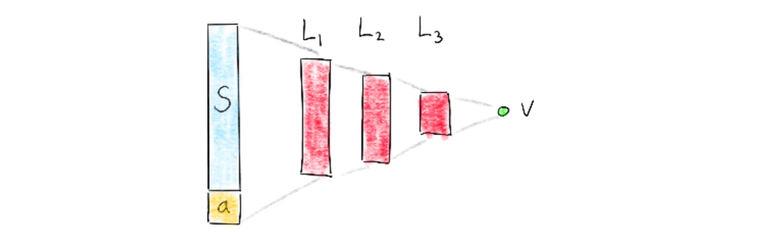#### Action Space in Output#### Action Space with Layer in Output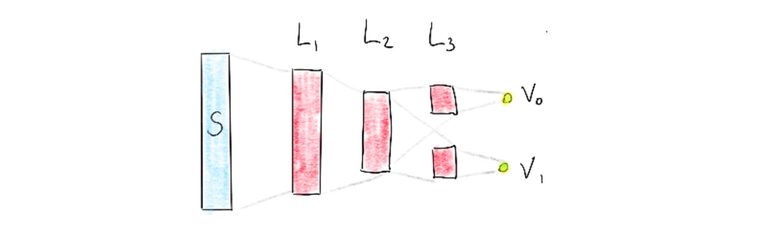The deepmind paper briefly discusses the architecture choice too. They used an architecture in which there is a separate output unit for each possible action and only the state representation is an input to the neural network. The article mentions learning performance as a reason for doing so but it also feels right to have the outputs make use of the same latent feature space that is generated in the network too.

There is another curious benefit. Suppose we have a $$(S_t, a_0, v)$$ triplet, then we'll only need to update weights that can influence the output of $$a_0$$. This has the benefit that the output nodes push and pull the weights of network in different methods. Potentially, this can lead to a nice latent feature representation.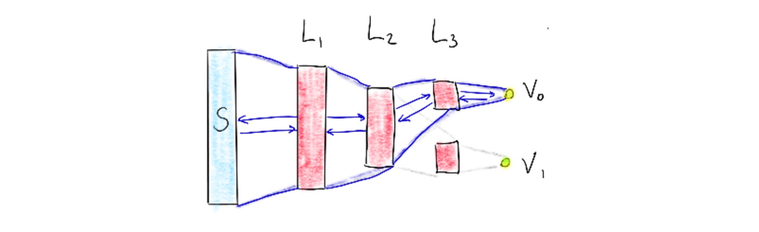I've chosen to implement this last network, more by intuition then anything else.

## Code

Turns out that writing such an architecture is relatively straightforward in keras. I'll list part of the code below.

from keras.models import Model
from keras.layers import Dense, Input, Dropout

outputs = []
main_input = Input(shape=(self.input_size,), name = "input")
mod = Dense(output_dim=10, input_dim=self.input_size , activation="relu")(main_input)
mod = Dense(output_dim=10, activation="relu")(mod)
self.base_model = mod
for i in range(self.output_size):
pre_output_layer = Dense(output_dim=10, activation="relu")(self.base_model)
output_node = Dense(output_dim=1, activation="linear")(pre_output_layer)
output_model = Model(input = main_input, output = output_node)
outputs.append(output_model)


This functional API allows you to be very flexible indeed.

## Some Results

The results are mixed it doesn't always seem to find a good strategy but when it does, I recognize an interesting pattern. I'll list two plots from two such examples.

•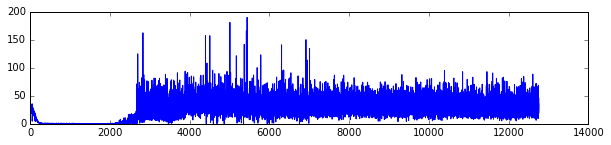•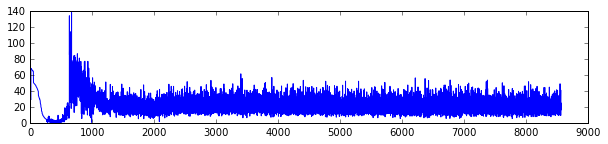•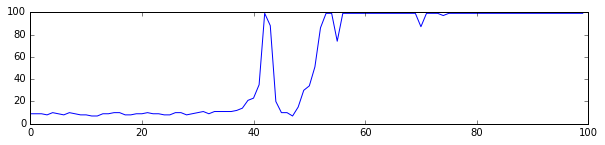•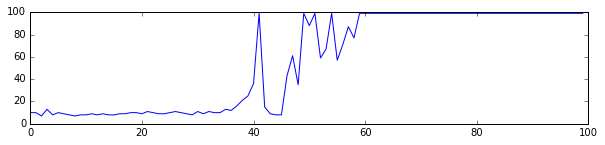•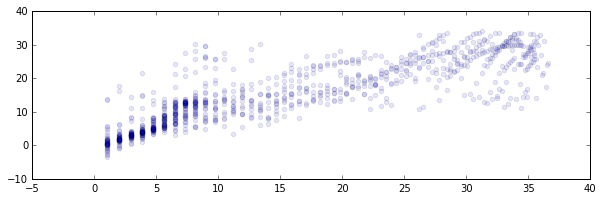•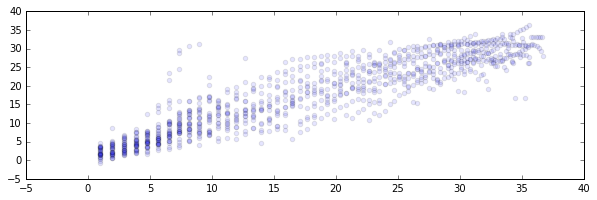For each run you'll see three plots listed;

• The loss rate of the (deep) neural network; you'll notice that the loss is very good initially and then suddenly spikes. The x-axis is a bit inconsistent because the training batch size depends on the number of $$(S, a, v)$$ triplets which depends on the performance of the agent. If the agent performs very well, there's longer lasting instances of games, which leads to more data. If the agent performs poorly then there's less data during an epoch.
• The performance of the agent; you'll notice that the performance initially is very poor, up until a moment where the agent (probably accidentally) performs very well. This is followed by a brief period of confusion after which the agent seems to be performing well.
• The prediction vs. true value of $$Q(S, a)$$. You'll notice that a lot of mass is around the low values but there seems a nice linear relationship between true value and predicted value.

In these plots I tend to see a similar pattern. Initially, the algorithm is very good at predicting the value; it will always perform poorly. Later, the robot accidentally did something well which causes the sudden spike. While trying to understand what it just did that caused the high score there is a brief moment of confusion but after a while it learns the correct action.

## Generality

This approach won't work for continous action spaces. But it is easy to come up with an alternative network representation to facilitate this.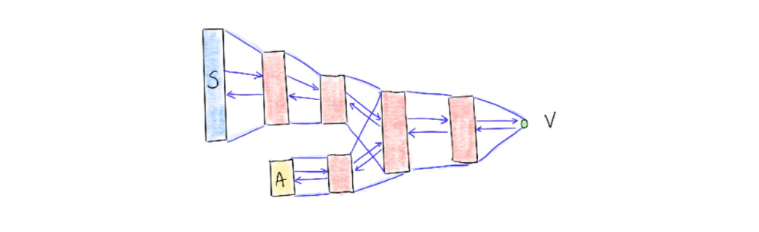This method of thinking about networks is relatively general which is why the research interest is so high in this area.

## Or maybe not

But this will only work in environments where you can sample and simulate. The gym environment from OpenAI is essentially giving me an infinite amount of data to learn from (and to make mistakes in). This isn't that realistic as a training procedure for real examples "out there".

## Conclusion

I'm attempting to write a few general python objects to tackle some open-ai problems. It's a bit new to me but if you're interested you'll find some code on github. My approach seems to show potential, but there still seems to be a lot of tweaking hyperparameters. An obvious one is the shape of the neural network but there's also things like:

• How many epochs do we use for learning?
• What value of $$\gamma$$ is appropriate?
• The agent sometimes can pick a random action to prevent getting stuck in a local optima ($$\epsilon$$ greedy, what value of $$\epsilon$$)?
• What activation functions made the most sense for the internal networks?
• Is it allright to forget previous trials? If the initial trials are usually achieved by noise, should the neural network consider this data as equal?
• Will this approach work for situations where the state space is only partially observable or will the noise cause too much distress for the NN?
• Do we want to have a deterministic policy, one that always takes the maximum value predicted by the NN or a stochastic policy?
• What is an appropriate learning rate for the neural network?
• While we're at it; what is an appropriate gradient method? SGD? Adam?

It is also worth noting that the DQL approach seems interesting beause it is general, but it is by no means the only way to do Q-learning. You can also use update rules or policy gradients.